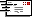Volumetric Analysis
version 2.1

[ Buy Volumetric Analysis • Also available: 16-bit version ]

Overview

Volumetric Analysis can be used:
• For titration problem drills,
• As a quick way to calculate titration solutions (with worked answers),
• To check your own answers in real titrations,
• By teachers to generate an almost unlimited number of titration problems for testing.

Using the Volumetric Analysis program• 3 Different Titration Types
• Acid-Base
• Oxidant-Reductant
• Iodine-Thiosulphate
• Select Reactants from the two dropdown boxes,
• Enter your own Data,
• Set which reactant is the standard solution
• or
• Randomly generate it:
•Randomly generate questions, with new settings for Titration Type, Reactants and data
•Randomly generate data for the current question settings
• Show or Hides the Data box or Question Area parts of the display
• Show Volume in mL and L or cmł and dmł
•Pop up the Windows calculator
•Show a worked solution to the problem using 4 step method

Solving Volumetric Analysis Problems

A Four Step process is used in the Volumetric Analysis Solution windowEach click of the Next Step button moves one step closer to answering the question

Step 1.  Balanced Equation(s) . This step is need to find the mole ratio used in step 3 (moles of unknown : moles of known)

Step 2.   moles of the "known" reagent, using n=cv (or sometimes n=m/M)

Step 3.   moles of the "unknown" reagent, worked out by using the first two steps,
i.e. mole ratio of (unknown / known) X moles of known

Step 4.    the final step (answering the question!), using step 3 and c=n/v (or sometimes m=nM) to calculate the concentration, or mass or percentage etc. of the unknown

Buy Volumetric AnalysisChemware Order FormDescription and Online Ordering at The Virtual Software Store[ Top ]

[ C h e m w a r e   S u i t e    C D - R O M ]

Electrochemical Cells [ Student Version | Pro Version | Free Download ]
Organic Chemistry [ Student Version | Free Download | Pro Version ]
[ Chemical Formula Tutor | Free Download ]
pH & Titration Curves [ 16-bit | 32-bit ]
Volumetric Analysis [ 16-bit | 32-bit ]webmaster@chemware.co.nz